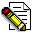## Firefly and PC GAMESS-related discussion clubLearn how to ask questions correctly

SCF convergence

Masoud Nahali
masoudnahali@gmail.com

Dear Firefly Users

My study system contains 174 atoms (C, H, and Ni) and as proposed by Sanya in the discussion with David G. , I have used 6-31g*, 6-31+g*, and Lanl2dz for C, H, and Ni respectively to overcome the possible linear dependence problem. After about 200 steps, the system was not converged. It seems that I must increase the MAXIT but it takes a long time to be converged. How can I increase the efficiency of the SCF procedure to get the convergence in a shorter time ?

These are the variables of my input file :

\$CONTRL SCFTYP=RHF RUNTYP=OPTIMIZE DFTTYP=B3LYP ECP=read MAXIT=200 MULT=1
ICHARG=0 COORD=unique d5=.t. NZVAR=516 \$END
\$SYSTEM TIMLIM=6897500000 MWORDS=200 \$END
\$SCF DIRSCF=.TRUE. NCONV=4.5 \$END
\$STATPT OPTTOL=0.0001 NSTEP=150 \$END
\$zmat dlc=.true. auto=.true. \$end
! to activate P2P inteface and DLB:
\$P2P P2P=.T. DLB=.T. \$END
! to speed up Huckel guess:
\$GUESS GUESS=HUCKEL KDIAG=0 \$END
\$smp call64=.t. mkl64=8 load=0 ssg32=0 msu32=0 mkllvl=3 \$end
\$BASIS gbasis=LANL2DZ extfil=.t. \$END

and the SCF steps was attached.This message contains the 20 kb attachment [ A.txt ]Sun Jan 23 '11 5:23pmThis message read 866 times